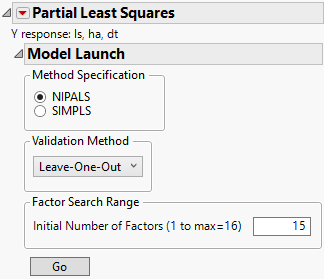Multivariate Methods > Partial Least Squares Models > Model Launch Control Panel
Publication date: 11/10/2021

# Model Launch Control Panel

After you click OK in the platform launch window (or Run in the Fit Model window), the Model Launch control panel appears.

Figure 6.8 Partial Least Squares Model Launch Control PanelNote: The Validation Method portion of the Model Launch control panel appears differently in JMP Pro.

The Model Launch control panel contains the following selections:

Method Specification

Select the type of model fitting algorithm. There are two algorithm choices: NIPALS and SIMPLS. The two methods produce the same coefficient estimates when there is only one response variable. See Statistical Details for the Partial Least Squares Platform for more information about differences between the two algorithms.

Validation Method

Select the validation method. Validation is used to determine the optimum number of factors to extract. For JMP Pro, if a validation column is specified on the platform launch window, these options do not appear.Holdback

Randomly selects the specified proportion of the data for a validation set, and uses the other portion of the data to fit the model. The random selection is based on stratified sampling across the model factors to attempt to create training and validation sets that are more balanced than ones based on simple random sampling.KFold

Partitions the data into K subsets, or folds. In turn, each fold is used to validate the model that is fit to the rest of the data, fitting a total of K models. This method is best for small data sets because it makes efficient use of limited amounts of data.

Leave-One-Out

Performs leave-one-out cross validation.

None

Does not use validation to choose the number of factors to extract. The number of factors is specified in the Factor Search Range.

Factor Search Range

Specifies how many latent factors to extract if not using validation. If validation is being used, this is the maximum number of factors the platform attempts to fit before choosing the optimum number of factors. The maximum number of factors is the minimum of the number of non-missing rows and the number of factors. Then, the initial number of factors is the minimum of 15 and the maximum number of factors.

Factor Specification

Appears once you click Go to fit an initial model. Specify a number of factors to be used in fitting a new model.

Go

Launches the model fit with the given specifications.